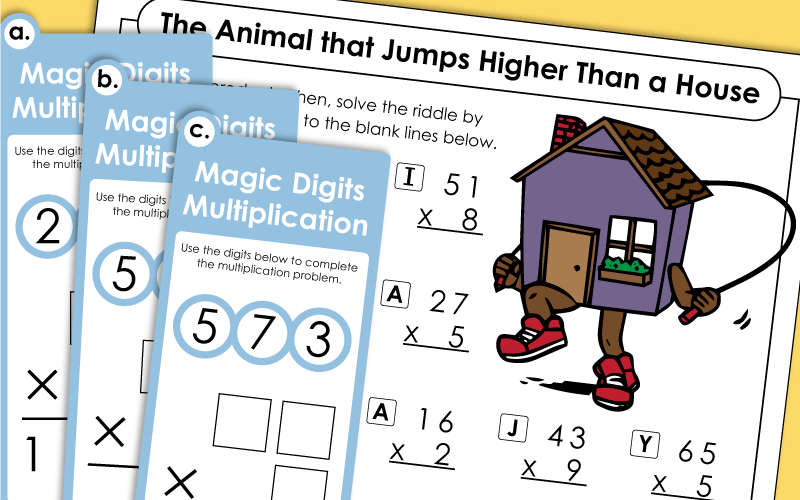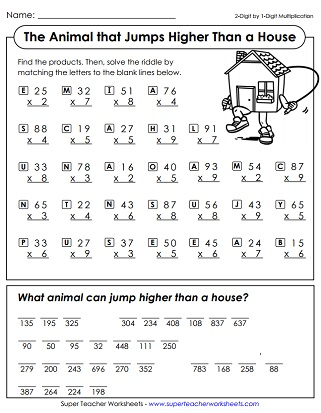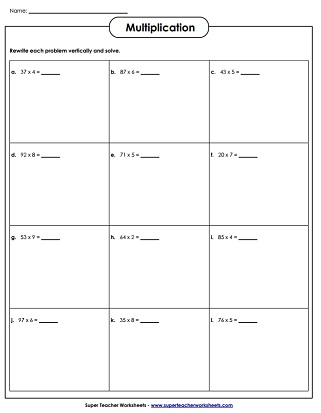# Multiplication Worksheets (2-Digit Times 1-Digit)

On this page you'll find a variety of printables for teaching 2-digit by 1-digit multiplication. We have math riddle worksheets, a multi-digit multiplication dice game, task cards, horizontal and vertical problem worksheets, and much more.## 2-Digit Times 1-DigitWorksheets

Solve the 2-digit by 1-digit multiplication problems. Then use the answers to decode the solution to a silly riddle. Why did the cow do jumping jacks? She wanted to make a milkshake.
This worksheets has several two-digit by one-digit multiplication word problems. (example: 56 x 3)
This worksheet has nine horizontal multiplication problems. Students rewrite each problem vertically and solve. (example: 54 x 6)
These completed multiplication problems have mistakes in them. Find and explain the error in each. Then resolve.
Solve eight 2-digit by 1-digit multiplication problems. This worksheets has pictures of cute monsters.
This variety worksheet features both horizontal and vertical problems, word problems, and a table.
Students are presented with shapes and symbols. They use the cypher key at the top of the page to decode the symbols into numbers. Then they multiply to solve.
On the top of this printable, students are presented with twelve shapes. Each one has a number in it. They multiply congruent shapes together. For example: Find the product of the numbers in the trapezoids.
Graph Paper Math Drills; 2-digits times 1-digit (example: 47 x 6)
Complete the math on this page, then use the products to answer a funny riddle at the bottom. Riddle says: What animal jumps higher than a house?
Use this worksheet to practice multiplying multiples of 10 by single-digit numbers.

Multiplication Worksheet Generator

Our generator tool allows you to make customized multiplication worksheets. Select whether you'd prefer vertical or horizontal problems. Then tell how many digits you'd like in each factor.

In this game, students will try to solve 12 tricky task cards. For each card, students must rearrange a set of digits to complete a multiplication problem.
Roll dice to make two-digit by one-digit multiplication problems. Then solve each one.
This is a set of 30 task cards that can be used for a variety of classroom activities, such as review games, study tools, and learning centers.

## Multiplication Patterns

Place value patterns in multiplication; Learn to use mental math to multiply large numbers that end in zero (example: 400 x 6)
More Multi-Digit Multiplication

Check out the full index page of multi-digit multiplication topics on Super Teacher Worksheets.

Basic Multiplication Facts

Review basic multiplication facts with the printable games and worksheets found here.

## Worksheet ImagesMy Account
Site Information